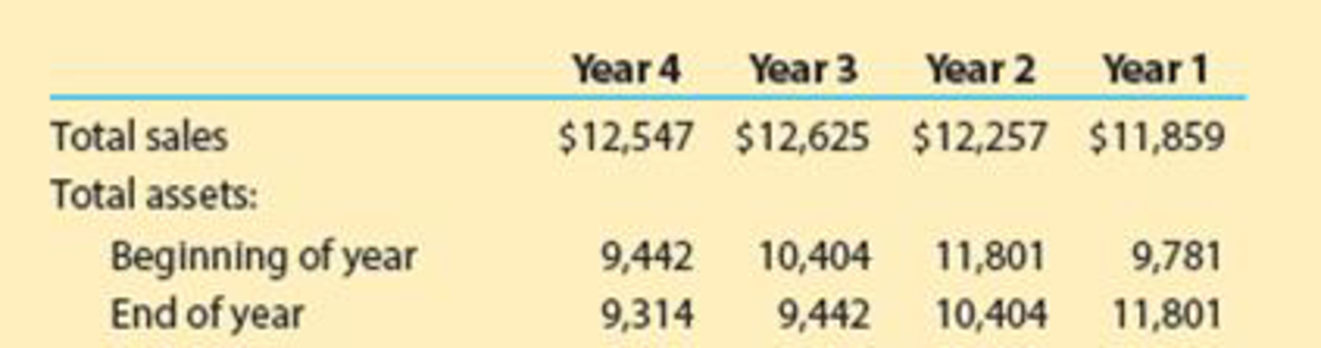# Analyze J. C. Penney J. C. Penney Company, Inc. (JCP) is a large general merchandise retailer in the United States. The following data (in millions) were obtained from its financial statements for four recent years: a. Compute the asset turnover ratio for each year. Round to two decimal places. b. Plot the asset turnover ratio on a line chart with the year on the horizontal axis. c. Interpret the trend in this ratio over the four years.### Financial And Managerial Accounting

15th Edition
WARREN + 1 other
Publisher: Cengage Learning,
ISBN: 9781337902663

#### Solutions

Chapter
Section### Financial And Managerial Accounting

15th Edition
WARREN + 1 other
Publisher: Cengage Learning,
ISBN: 9781337902663
Textbook Problem
19 views

## Analyze J. C. PenneyJ. C. Penney Company, Inc. (JCP) is a large general merchandise retailer in the United States. The following data (in millions) were obtained from its financial statements for four recent years:a.    Compute the asset turnover ratio for each year. Round to two decimal places.b.    Plot the asset turnover ratio on a line chart with the year on the horizontal axis.c.    Interpret the trend in this ratio over the four years.

a.

To determine

Calculate the asset turnover ratio of Company JCP for the four years.

### Explanation of Solution

Asset turnover ratio: Asset turnover ratio measures the productive capacity of the total assets to generate the sales revenue for the company. Thus, it shows the relationship between the net sales and the average total assets.

Calculate the asset turnover ratio for Year 1.

Asset turnover ratio = TotalsalesAverage assets=Totalsales(Assets at the beginning of the year+Assets at the end of the year)2=$11,859($9,781+$11,8012)=1.10 times Calculate the asset turnover ratio for Year 2. Asset turnover ratio = TotalsalesAverage assets=Totalsales(Assets at the beginning of the year+Assets at the end of the year)2=$12,257($11,801+$10,4042)=1

b.

To determine

Prepare a line chart with the year on the horizontal axis for the asset turnover ratio.

c.

To determine

Interpret the trend of the asset turnover ratio of Company JCP over the four years.

### Still sussing out bartleby?

Check out a sample textbook solution.

See a sample solution

#### The Solution to Your Study Problems

Bartleby provides explanations to thousands of textbook problems written by our experts, many with advanced degrees!

Get Started